#### 期刊菜单

Design of Visual Real-Time Rendering Engine
DOI: 10.12677/SEA.2022.113062, PDF , HTML, XML, 下载: 187  浏览: 426  科研立项经费支持

Abstract: The rendering engine encapsulates image rendering and other related technologies, and provides a unified interface for developers to develop image programs. Through the research of 3D real-time rendering, a fast and efficient visual rendering engine is developed by using physics based rendering, multi sampling anti aliasing and other technologies. It realizes the functions of automatic model loading, multi-threaded rendering, real-time adjustment of rendering picture settings and so on. The rendering engine can be applied to film special effects production, 3D animation rendering and other fields.

1. 引言

2. 抗锯齿的渲染引擎

2.1. 辐射度量学Figure 1. Solid angle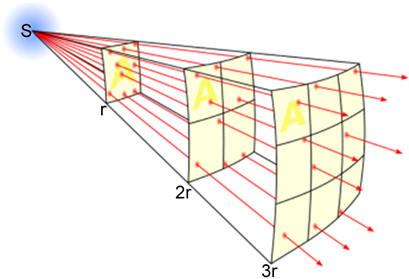Figure 2. Radiation proportion at different distances

2.2. 双向反射分布函数(Bidirectional Reflectance Distribution Function, BRDF)

${k}_{d}\frac{c}{\pi }$ (1)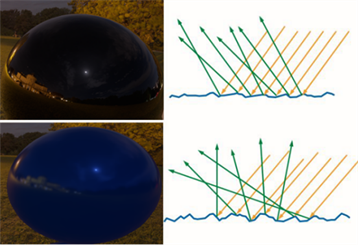Figure 3. Contrast of different normal concentration

${f}_{r}={\kappa }_{d}{f}_{\text{lambert}}+{\kappa }_{s}{f}_{\text{cook-torrance}}$ (2)

${f}_{r}=\frac{1}{\pi }$。在考虑上反射率之后，即可得到最终的漫反射BRDF：

${f}_{\text{lambert}}=\frac{\rho }{\pi }$ (3)

${f}_{\text{cook-torrance}}=\frac{F\left(l,h\right)G\left(l,v\right)D\left(h\right)}{4\left(n\cdot l\right)\left(n\cdot v\right)}$ (4)

$\begin{array}{l}0\le G\left(l,v\right)\le 1\\ 0\le F\left(l,h\right)\le 1\end{array}$ (5)

3. 实验结果与分析

3.1. 抗锯齿渲染效果Figure 4. Anti aliasing example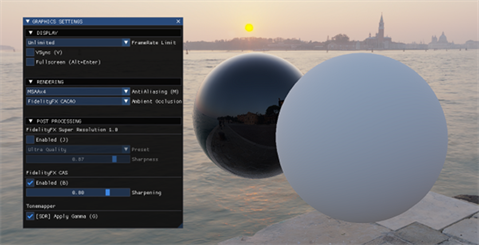Figure 5. Anti aliasing module

3.2. 天空盒渲染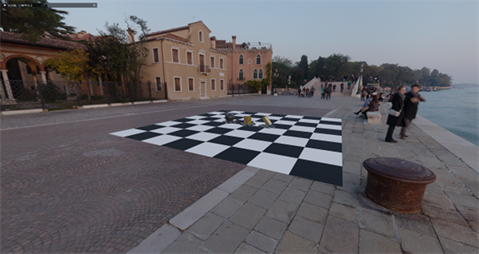Figure 6. Sky box renderings

3.3. 基于图像的光照渲染

3.4. 基于物理的渲染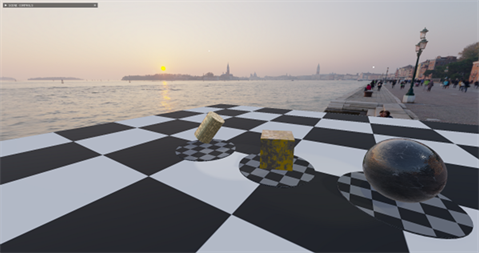Figure 7. Image based lighting example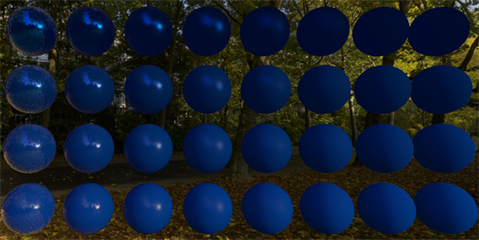Figure 8. Rendering of different normal distribution functions

4. 结论

NOTES

*通讯作者。

  李远航. 三维光场显示实时编码与渲染方法研究[D]: [博士学位论文]. 北京: 北京邮电大学,2021.  孟令增, 王中训. 基于PBR的渲染算法研究[J]. 电声技术, 2021, 45(11): 58-60.  曹天池, 李秀实, 李丹, 陈嘉南, 刘爽, 向未来, 胡迎松. 基于图像分解的光照估计算法[J]. 计算机工程与科学, 2021, 43(8): 1422-1428.  张宇剑. 图形处理器三角形光栅化和抗锯齿算法研究与实现[D]: [硕士学位论文]. 西安: 西安电子科技大学, 2018.  景志敏. 基于GPU的矢量地图连续LOD渲染方法研究[D]: [硕士学位论文]. 南京: 南京师范大学, 2021.  李远航. 三维光场显示实时编码与渲染方法研究[D]: [博士学位论文]. 北京: 北京邮电大学,2021.  孟令增, 王中训. 基于PBR的渲染算法研究[J]. 电声技术, 2021, 45(11): 58-60.  曹天池, 李秀实, 李丹, 陈嘉南, 刘爽, 向未来, 胡迎松. 基于图像分解的光照估计算法[J]. 计算机工程与科学, 2021, 43(8): 1422-1428.  张宇剑. 图形处理器三角形光栅化和抗锯齿算法研究与实现[D]: [硕士学位论文]. 西安: 西安电子科技大学, 2018.  景志敏. 基于GPU的矢量地图连续LOD渲染方法研究[D]: [硕士学位论文]. 南京: 南京师范大学, 2021.